# How to color the vertices of a graph

In this short note, I want to show how, using algebra, you can solve the classical problem of coloring the vertices of a graph. I learned about this story from a book. W. W. Adams, P. Loustanau. An Introduction to Groebner Basis (Section 2.7).

## Introduction

First, let’s discuss all the necessary concepts. Letis a set, andis a set consisting of unordered pairsset elements. Then count called a coupleWhereincalled many peaks graph, andmany edges graph. Peakscalled relatedif they are connected by an edge, that is.

Consider a graph consisting of three vertices, which are pairwise connected by edges. The set of vertices of such a graph has the formand the set of edges. All vertices of a graph are adjacent. It is convenient to imagine this graph by drawing it on a plane.

Note that in doing so, we were able to draw the edges so that they do not intersect outside the vertices. Graphs for which this can be done are called planar or flat. Not every graph is planar. For example, a graph with five vertices, in which every pair of distinct vertices is adjacent, will not be planar.

Let– set ofelements (many colors). Then vertex coloring countviacolors is called mappingsuch that for any pairadjacent vertices of the graph, the condition. In other words, to each vertex we uniquely associate one ofcolors in such a way that the vertices connected by an edge are painted in different colors. For simplicity, we will consider only the case of three colors (), although the methods used are suitable for any. Our three-vertex graph can be easily colored with three colors.

So, we want to determine from a graph whether it is possible to color its vertices in three colors, so that adjacent vertices are not colored in the same one.

## Algebra comes to the rescue

Let a pair of setsandgiven graph. The main idea is to associate our graph with a system of algebraic equations. As a set of colors we will use the set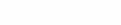whereThis set consists of cube roots of unity, that is, such complex numbers that, when raised to the third power, give(in the general case, you need to consider the set of roots-th degree). We will assume that each vertexis a variable. When coloring, each such variablecan take one of the valuesWe can express this fact in algebraic form as follows: when choosing one of the three indicated values ​​of the variablethe polynomial must vanish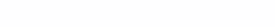Thus, we get a system fromalgebraic equations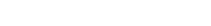However, for now, we do not take into account the fact that adjacent vertices cannot be painted in the same color. emptyandare adjacent vertices, that is, the setcontains an edgeThen the equality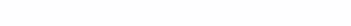Since the vertices are adjacent, we use different colors for them, which means. Consequently, the first factor in the above product cannot be equal to zero, which means that the second bracket must go to zero. Thus, to the existingequations, we must add an equation of the form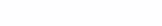for each edge. Now the question of coloring the vertices of the graph has turned into the question of the compatibility of a system of algebraic equations, that is, the question of the existence of a solution for such a system. If the system has no solutions, then the graph cannot be colored. If solutions exist, then each gives a way to color the vertices of the graph.

Readers familiar with commutative algebra know that this question of the compatibility of a system of algebraic equations over an algebraically closed field is solved with the help of the so-called weak Hilbert theorem on zeros and the theory of Gröbner bases. In the next paragraph, we will use the function of solving systems of equations built into the SciPy module to implement the parsed method in Python.

## Implementation in Python

As a library that allows you to work with graphs, we will use igraph.

We will test our script on the next graph of eight vertices. Note that this graph is planar, although Kawada and Kawai’s algorithm could not lay it flat without crossing edges outside the vertices.

``````from igraph import *
from sympy import solve, symbols

# Зададим количество вершин
NumberOfVertices = 8
# Перечислим все ребра нашего графа
EdgesList = [(0,1), (0,4), (0,5),  (1,7), (1,2), (2,3), (2,7), (1,3), (3,4), (3,6), (4,5), (4,6),(5,6), (6,7)]

# Инициализируем граф, обозначив его вершины с помощью символов x1,...x8
TestGraph = Graph()
x1, x2, x3, x4, x5, x6, x7, x8 = symbols("x1 x2 x3 x4 x5 x6 x7 x8")
TestGraph.vs["name"] = [x1, x2, x3, x4, x5, x6, x7, x8]
TestGraph.vs["label"] = TestGraph.vs["name"]

# Генерируем уравнения для системы, определяющей раскраску
EquationList=[]
for edge in EdgesList:
EquationList.append("x%d^2 + x%d * x%d + x%d^2"%(edge+1,edge+1,edge+1,edge+1))
for vertice in range(NumberOfVertices):
EquationList.append("x%d^3-1"%(vertice+1))

# Сопоставляем кубическим корням из единицы красную, зеленую и синию краски
Roots = solve(x1**3-1)
RootsToColors = {Roots: "red", Roots: "green", Roots: "blue"}

# Непосредственно решаем систему уравнений
Colorings = solve(EquationList, dict=True)
print("The number of colorings is %d."%len(Colorings))

# Если система совместна, то выводим k-ю раскраску.
# Если нет, то делаем вывод о том, что граф нельзя раскрасить в три цвета.
if(Colorings):
# Раскрашиваем вершины графа
k = 0
RawColors = [Colorings[k][vertice] for vertice in TestGraph.vs["name"]]
ColorDictionary = [RootsToColors[color] for color in RawColors]
TestGraph.vs["color"]=ColorDictionary

# Укладываем граф на плоскость и рисуем
visual_style = {}
visual_style["vertex_size"] = 40
visual_style["bbox"] = (300, 300)
plot(TestGraph, layout=Layout, **visual_style)
else:
print("The graph is non-colorable.")``````

As a result, we obtain one of the possible colorings.

However, the coloring for this graph is not difficult to obtain without any science, by trial and error. However, adding to the graph an edge connecting the verticesand, we arrive at a graph that cannot be colored. In this case, the trial and error method is powerless.